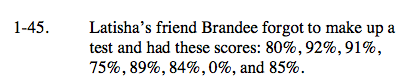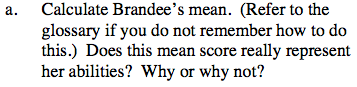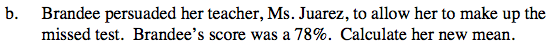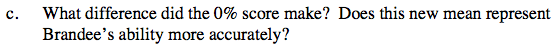### Home > CAAC > Chapter 1 > Lesson 1.2.1 > Problem1-45

1-45.The average is the sum of all the scores divided by the number of scores.

$\frac{80+92+91+75+89+84+0+85}{8}=74.5\%$

Compare this average with her scores. Does it seem right? Why or why not?See part (a) for the definition of average.

$\frac{80+92+91+75+89+84+75+85}{8}=84.25\%$How did a 75% instead of a 0% change her average? Does it seem like the new average is better? How?